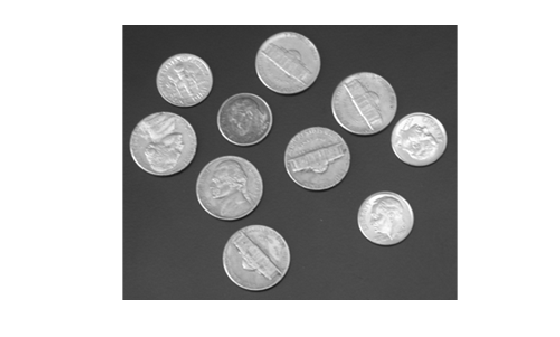## Edge Detection

In an image, an edge is a curve that follows a path of rapid change in image intensity. Edges are often associated with the boundaries of objects in a scene. Edge detection is used to identify the edges in an image.

To find edges, you can use the `edge` function. This function looks for places in the image where the intensity changes rapidly, using one of these two criteria:

• Places where the first derivative of the intensity is larger in magnitude than some threshold

• Places where the second derivative of the intensity has a zero crossing

`edge` provides several derivative estimators, each of which implements one of these definitions. For some of these estimators, you can specify whether the operation should be sensitive to horizontal edges, vertical edges, or both. `edge` returns a binary image containing 1's where edges are found and 0's elsewhere.

The most powerful edge-detection method that `edge` provides is the Canny method. The Canny method differs from the other edge-detection methods in that it uses two different thresholds (to detect strong and weak edges), and includes the weak edges in the output only if they are connected to strong edges. This method is therefore less likely than the others to be affected by noise, and more likely to detect true weak edges.

### Detect Edges in Images

This example shows how to detect edges in an image using both the Canny edge detector and the Sobel edge detector.

```I = imread('coins.png'); imshow(I)```Apply both the Sobel and Canny edge detectors to the image and display them for comparison.

```BW1 = edge(I,'sobel'); BW2 = edge(I,'canny'); figure; imshowpair(BW1,BW2,'montage') title('Sobel Filter Canny Filter');```